### IMO Shortlist 1997 problem 8

Kvaliteta:
Avg: 3,0
Težina:
Avg: 6,0
It is known that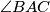$\angle BAC$ is the smallest angle in the triangle$ABC$. The points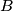$B$ and$C$ divide the circumcircle of the triangle into two arcs. Let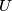$U$ be an interior point of the arc between$B$ and$C$ which does not contain$A$. The perpendicular bisectors of$AB$ and$AC$ meet the line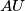$AU$ at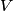$V$ and$W$, respectively. The lines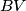$BV$ and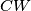$CW$ meet at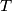$T$.

Show that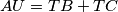$AU = TB + TC$.

Alternative formulation:

Four different points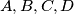$A,B,C,D$ are chosen on a circle$\Gamma$ such that the triangle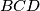$BCD$ is not right-angled. Prove that:

(a) The perpendicular bisectors of$AB$ and$AC$ meet the line$AD$ at certain points$W$ and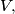$V,$ respectively, and that the lines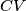$CV$ and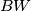$BW$ meet at a certain point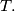$T.$

(b) The length of one of the line segments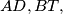$AD, BT,$ and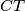$CT$ is the sum of the lengths of the other two.
Izvor: Međunarodna matematička olimpijada, shortlist 1997